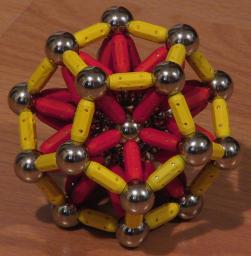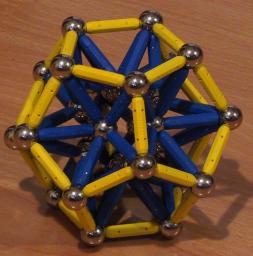# Platonic Solids

Platonic solids are convex polyhedra with equivalent faces, which are regular polygons. There are only five of them, and they are all fairly easy to build.

## Tetrahedron

The tetrahedron is composed out of 4 triangular faces. It has 4 vertices and 6 edges. Since its triangular edges are stable without support, 4 spheres and 6 bars are sufficient for its construction.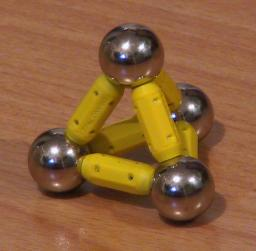## Octahedron

The octahedron is composed out of 8 triangular faces. It has 6 vertices and 12 edges. Since its triangular edges are stable without support, 6 spheres and 12 bars are sufficient for its construction.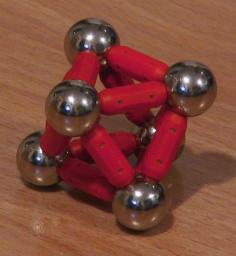## Icosahedron

The icosahedron is composed out of 20 triangular faces. It has 12 vertices and 30 edges. Since its triangular edges are stable without support, 12 spheres and 30 bars are sufficient for its construction.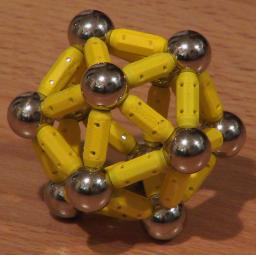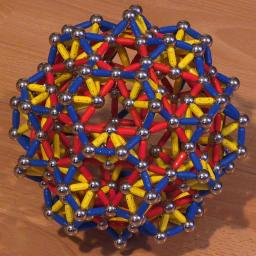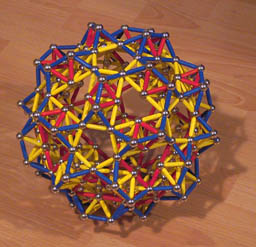The second and third icosahedrons here use a bridge-like structure for its edges. See my page on scaling polyhedra for details.

## Cube

The cube is composed out of 6 square faces. It has 8 vertices and 12 edges. The square faces need support, for which there are two simple options: Use small bars for the edges and stabilize each face with a long bar as a diagonal. For this construction, 8 spheres, 12 short bars, and 6 long bars are needed. The other option is to use long bars for the edges and to support the faces with 4-star built out of one sphere and 4 short bars each. This construction requires 12 spheres, 12 long bars, and 24 short bars.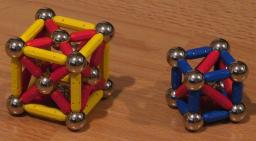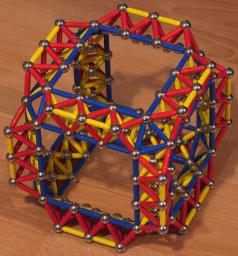The cube in the second picture uses a bridge-like structure for its edges. See my page on scaling polyhedra for details.

## Dodecahedron

The dodecahedron is composed out of 12 pentagonal faces. It has 20 vertices and 30 edges. The easiest way to build it is to stabilize each face with a 5-star. Such a construction requires 32 spheres and 90 bars.